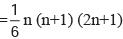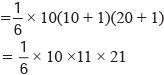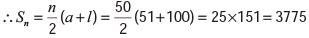Courses

Olympiad Test: Number System - 3

10 Questions MCQ Test Mathematics Olympiad Class 7 | Olympiad Test: Number System - 3

Description
Attempt Olympiad Test: Number System - 3 | 10 questions in 20 minutes | Mock test for Class 7 preparation | Free important questions MCQ to study Mathematics Olympiad Class 7 for Class 7 Exam | Download free PDF with solutions
QUESTION: 1

What will be the value of (12 + 22 + 32 + ... + 102) = ?

Solution:

We know that (12 + 22 + 32 + ... + n2)Putting n=10, required sum= 385

QUESTION: 2

What will be the sum of (51+ 52 + 53 + ... + 100) = ?

Solution:

This is an A.P. in which a = 51, l = 100 and n = 50.QUESTION: 3

What will be the last digit in (795– 358)?

Solution:

Unit digit in 795 = Unit digit in [(74)23 × 73]
= Unit digit in [(Unit digit in(2401))23 × (343)]
= Unit digit in (123 × 343) = Unit digit in (343) = 3
Unit digit in 358 = Unit digit in [(34)14 × 32]
= Unit digit in [Unit digit in (81)14 × 32]
= Unit digit in [(1)14 × 32]
= Unit digit in (1 × 9)
= Unit digit in (9) = 9
Unit digit in (795 – 358)
= Unit digit in (343 – 9)
= Unit digit in (334) = 4.

QUESTION: 4

Find the value of 1904 × 1904 = ?

Solution:

904 × 1904 = (1904)2
= (1900 + 4)2
= (1900)2 + (4)2 + (2 × 1900 × 4)
= 3610000 + 16 + 15200
= 3625216

QUESTION: 5

The least six digit number completely divisible by 111 is:

Solution:

The least six digit number is 100000.
When 100000 ÷ 111,
Quotient 990 and Remainder = 100
Therefore required number
=100000 + ( 111 – 100)
= 100000 + 11
= 100011

QUESTION: 6

Find the number of terms in the given G.P. series 3, 6, 12, 24... 384.

Solution:

Here a = 3 and r =6/3 =2.
Let the number of terms be n.
Then, tn = 384
arn-1 = 384
3 × 2n – 1 = 384
2n-1 = 128 = 27
n – 1 = 7
n = 8
Number of terms = 8.

QUESTION: 7

What will be the largest 5 digit number completely divisible by 91?

Solution:

Largest 5-digit number = 99999
When 99999÷91, Quotient = 1098 and
Remainder = 81
Required number = (99999 – 81)
= 99918.

QUESTION: 8

When we multiply a certain number by 7, we obtain product whose each digit is 3. What will be that number?

Solution:

By hit and trial, we find that
47619 × 7 = 333333.

QUESTION: 9

If 25% of  2/5 of a certain number is 125 then the required number is:

Solution:

Let the required number be n,
Then 25% × 2/5 × n = 125
By solving the above equation,
∴ n = 1250

QUESTION: 10

Which of the following cannot be the square of counting number?

Solution:

The square of a natural number never ends in 7.
42437 is not the square of a natural number.Use Code STAYHOME200 and get INR 200 additional OFF Use Coupon Code

Track your progress, build streaks, highlight & save important lessons and more!

Similar ContentRelated tests